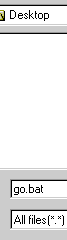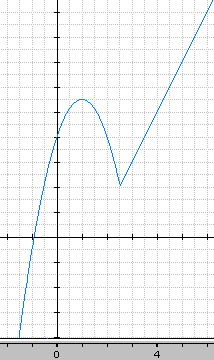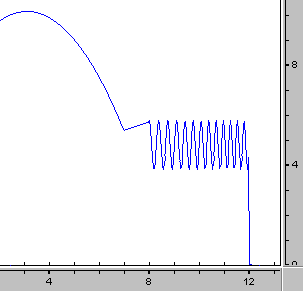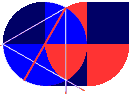Freeware

 Home | Freeware | Formula | Polygon | Books | LinksCreate you own computer program.
```      A program to search the WEB.

You can't download this program but I think that you are a
programmer who is much clever than those at "Mickey soft"!
So, just make your own program. Use it or create a gift to
`````` :: 1. Mouse! Move to the beginning of this line and click the left button. :: 2. Mouse! Move over the following lines. :: 3. Mouse: (tell your master that she can now release your left button). :: :: 4. Mouse! Click the right button and select COPY. :: :: 5. Mouse! Please open Notepad. (Click Start, Run and write Notepad) OK. :: :: 6. Mouse! Click the right button, select INSERT. :: :: 7. Mouse! Click on FILES (menu in the upper left corner). Click on SAVE :: 8. You may now write the following: GO.BAT :: 9. Mouse! Look down in the "save menu" and goto select: All files (*.*) :: SAVE. :: 10. Close Notepad: Just hold the ALT key and press the F4 key. FINISHED! ``` ```@echo off set TERMS=%1 :Loop if (%2) == () goto Ready set TERMS=%TERMS%+%2 shift goto Loop :Ready START http://www.google.com/search?q=%TERMS%&btnG=Google+Search&hl=en&lr=&safe=off set TERMS= ``` ``` :: Usage: GO word1 word2 and so on... :: Example: GO interesting DOS Laura :: Note: No words are necessary/required. :: :: * End of GO.BAT * A program to search the WEB * Written by YOUR NAME HERE :: * Additional information about GO.BAT by Gary L. Smith (Columbus, Ohio) * :: * If your new freeware program wont work, then you may try the following: :: * Move the file to "C:\Windows\Command\GO.BAT" or follow the above links. :: If you are able to copy and paste, then you can try this/another example. ```

 *  No math please!  Jump to next paragraph. November 27 - 2000 Off topic. -- It's time to publish my research.(Graph+ is updated February 18, 2001) Draw your math.   Download Graph+After an introduction (successful formulas to figure out Pi and things like that, I almost got a headache). I did that years ago. At that time I wanted to publish my math but I didn't (I got a computer to play with and so on...) The picture on the left shows one of my better math discoveries (I think?). You can put as many different formulas as you wish, together in just one formula and you can also decide where the next formula takes over, etc... Yesterday I was searching for a program to draw graphs and I was lucky to find the Graph+ freeware program. But frankly speaking I don't have much education about English and mathematics, etc.

 Here's an example of how to create a "X isn't 3" statement: Texas Ti-68 (my old calculator) -2-1 * (sig(-abs(x-3))-1) Computer (DOS) if not %X%==3 batch Graph+   |3-x|^(1/999)  Copy & paste. Zoom in: (x,y)=(3,1)
```
Note: the above |3-x|^(1/999), abs(3-x)(1/99999) is better but you'll miss the point.
```

Definition of sig(x)
```
x < 0    x = 0    x > 0
sig(x)       -1        1        1

```

BTW.: We maybe could find some possibilities in the programming of computers and take advantage of DOS batch, JavaScript, VBScript and HTML, or DHTML (Dyn HTML, JScript and DOM), etc... Anyhow, it is very exciting stuff...
```<a style="cursor:hand; text-decoration:underLine; color:#E0E0C0" onClick="JavaScript:
alert('\t' + +true + '\n\t' + +false)// Space: + +true, NOT ++true">
```
```<a style="cursor:hand; text-decoration:underLine; color:#E0E0C0" onClick="VBS:
msgBox vbTab & --true & vbCr & vbTab & --false,, 'True and False.'">
```

Definition of n, z and p

Let's say that n represents numbers less than 0, z represents zero
and p represents numbers greater than 0,
then according to the definition of sig(x),
sig(n) = -1 and sig(z) = sig (p) = 1

Now I say that the following is true:

h(x) = (sig(b-x) -sig(a-x)) / 2
h(x) = 0 | x <= a or x > b
h(x) = 1 | a < x <= b

And now I show you that it's true:

x can only satisfy one of the following five conditions:
less than a, equal to a, range between a (not included) and b
(not included), equal to b, or greater than b:
```
x1 x2 x3 x4 x5
----|--|--|--|--|---------->x
a     b
a < b
```
if x=x1, then both a and b are greater than x
so, according to the definition of p, both
(b-x) and (a-x) are equal to p:

h(x) = (sig(b-x) -sig(a-x)) / 2
h(x) = (sig(p) -sig(p)) / 2
h(x) = 0 = 0 / 2 = (1 -1) / 2

Now my assertion: h(x) = 0 | x <= a or x > b
is obviously true for x=x1.

if x=x2, then x=a and b is greater than x:

h(x) = (sig(p) -sig(z)) / 2

Since sig(z) = sig(p) and according to the above
demonstration for x=x1, then h(x) = 0 and since
x <= a or x > b is true, then my assertion is true for x=x2.

if x=x3, then a < x and b > x
h(x) should be 1. Let's see if that's true:

h(x) = (sig(p) -sig(n)) / 2
h(x) = 1 = 2 / 2 = (1 -(-1)) / 2

if x=x4, then a < x and b = x

h(x) = (sig(b-x) -sig(a-x)) / 2
h(x) = (sig(z) -sig(n)) / 2
h(x) = 1 = 2 / 2 = (1 -(-1)) / 2

and my assertion: h(x) = 1 | a < x <= b
is true for x=x4.

if x=x5, then a < x and b < x

h(x) = (sig(n) -sig(n)) / 2
h(x) = 0 = 0 / 2 = (-1 +1) / 2 = (-1 -(-1)) / 2

Conclusion: (tested and proved)
```
h(x) = (sig(b-x) -sig(a-x)) / 2
h(x) = 0 | x <= a or x > b
h(x) = 1 | a < x <= b

```
I would be surprised if my formula wasn't true for any value of x.
because I have used my system in practice/(real life).

About 10 years ago I was working in the post office at night.
I had to write a bill to those who hadn't put enough stamps on
their mail.

To figure out how many stamps to put on a letter, I had to take a look
at a table. Depending on (x), how many kilo or gram, (or if I didn't like that person:)
I wrote a big bill to pay for the missing stamps along with charges :-)

I had just got myself a pocket calculator. But to use it for this job was impossible. A big sour guy (the boss) didn't want me to use the pocket calculator because: everything should be done the way it always had been. Anyhow, like my boss: my pocket calculator couldn't figure out anything much and it was also impossible to program it except for simple arithmetic as plus or minus and so on. But I decided to overcome this difficulty and I devised my formulas. Moreover, I realized that my formulas was useful for any presentment of my mathematical problems.

Here's my "post formula":
```

f1(x) + fn(x)    n-1  fi+1(x) - fi(x)
F(X) = ------------- + SUM --------------- sig(x-bi)
2          i=1        2

fi(x)
|                   ---------    f4(x) | b3 <= x
|              -----             f3(x) | b2 <= x < b3
|      --------                  f2(x) | b1 <= x < b2
--|------                          f1(x) | b1 > x
_|____________________________x
|      b1      b2   b3

```
I told my friends that it's possible to draw a graph of a formula.
For example y=x, no surprise but when I said that I was able to put
all the different sort of formulas together in one formula and then all
I had to do was to hit a button on my pocket calculator, then they
just got a strange look on their face.
Even my math teacher thought that this was completely impossible.

Benny Pedersen
PS.
(((-(-40) +x)^(1/999) -(-(2.519) +x)^(1/999))/2) (-3/2(x^2) +3x +4) +(((-(2.519) +x)^(1/999) -(-(40) +x)^(1/999))/2) (2x -3)

It's the drawing you saw on the picture (-1.5 (x^2) +3x +4) and (2x -3)
(try substitute -40 with -3 or the second 2.519 with 4)

How to construct any formula in 10 seconds:

If you know a monkey? then let it paint a picture for you and when it's finished, then you can show your friends a formula to draw the graph of that painting.
Let's say the monkey painted a banana and then he (by an accident), dropped the paint brush.

To be continued...

```Raise abs(n) to the k'th power: |n|^(1/99999)
01
x = a
(1/2 +sig(-abs(a-x))/2) ~
(1 -(|a-x|^k))

02
x not a
(1/2 -sig(-abs(a-x))/2) ~
(|a-x|^k)

03
x < a
(1/2 -sig(x-a)/2) ~
((((a-x)^k)+(|a-x|^k))/2)

04
x <= a
(1/2 +sig(a-x)/2) ~
((((a-x)^k)-(|a-x|^k)+2)/2)

05
x >= a
(1/2 +sig(x-a)/2) ~
((-((a-x)^k)-(|a-x|^k)+2)/2)

06
x > a
(1/2 -sig(a-x)/2) ~
((-((a-x)^k)+(|a-x|^k))/2)

07
a <= x < b
(sig(x-a)/2 -sig(x-b)/2) ~
((-((a-x)^k)-(|a-x|^k)+((b-x)^k)+(|b-x|^k))/2)

08
a < x <= b
(-sig(a-x)/2 +sig(b-x)/2) ~
((-((a-x)^k)+(|a-x|^k)+((b-x)^k)-(|b-x|^k))/2)

09
a <= x<= b
(sig(x-a)/2 +sig(b-x)/2) ~
((-((a-x)^k)-(|a-x|^k)+((b-x)^k)-(|b-x|^k)+2)/2)

10
a < x < b
(-sig(a-x)/2 -sig(x-b)/2) ~
((-((a-x)^k)+(|a-x|^k)+((b-x)^k)+(|b-x|^k)-2)/2)

```

 ```Formula: n F(x) = SUM 1/2 fi(x) ( -((bi-1-x)k) +((bi-x)k) ) | b0 < x < bn i=1 Example: (n=3) f1(x) = -1/PI x2 +2x +7 | 2 < x <= 7.006978 | b0 = 2 f2(x) = 1/3 x +3.05 | 7.006978 <= x<= 8 | b1 = 7.006978 f3(x) = sin(x2) +4.8 | 8 <= x < 12.00001 | b2 = 8 b3 = 12.00001``` `F(x) | 2 < x < 12.00001````Graph+, copy and paste: ``` The following FAILS with Netscape, which don't understand HTML! ((-(Pi^(-1))(x^2)+2x+7) (-((2-x)^(1/99999))+((7.006978-x)^(1/99999))) + (    1/3 x +3.05     ) (-((7.006978-x)^(1/99999))+((8-x)^(1/99999))) + (    sin(x^2) +4.8   ) (-((8-x)^(1/99999))+((12.00001-x)^(1/99999))))/2 `Note: (don't miss the point) You don't see three formulas but ONLY one formula!```` Homemade polygon formula | (n > 2) Example n = 3 ``` ``` Radians: Polygon_around = n d sin(Pi/n) Polygon_area = Polygon_around 1/4 d cos(Pi/n) = 1/8 n d^2 sin(2 Pi/n) Degrees: Polygon_around = n d sin(180/n) Polygon_area = Polygon_around 1/4 d cos(180/n) = 1/8 n d^2 sin(360/n) ```
```

0 +1 +2 +3 +n +3 +2 +1 +0 = n^2
so therefore:
1 +2 +3 +n = ((n +1)^2 -(n +1))/2 = (n^2 +n)/2

```
End here at Dec 11, 2000,
(going to sleep)

GifOptimizer.
I havn't seen a better Gif optimizer than this one!

EjectCD.zip. EjectCD.bat is tested in Win98.
Author myself.

FTP.VBS A small freeware FTP program.
Author myself.

MultiClip.bat Win9X. A DOS batch file that you'll use very often.
(If you can't Copy Paste, see the notes along with the picture).

defragShutDown.zip Win 98/XP. Defrag (maintenance your PC), then shuts down your computer.

Clickie Win 95/98/2000, NT 4.0 or later. Two new right click actions:
[Copy PATH Name] or [Open MS-DOS Prompt (on folders).]

CdTree 1.1.5 is released. It creates a catalog of the files and folders on CDs.
vbKnowledgeSolutions.com

I found a link to a freeware FAX program. (Win95/98/NT/2000).
The VB6 runtime files is needed.

Directory Snoop is updated. A Win9x utility to undo deleted files: (recover, page 2)

Are your newly burnt CDROM okay?
CDCheck (Win95/98/NT) -- Checks the integrity of your data CDROMs and tells
you which files are corrupted. It can also check old CDROMs but not audio CDs.

Other Links: Recover - page 2, (Clone CD and CDCheck 3.0.0.27).

• What MP3 player should I use?

A comparison of the newest MP3 players on the Internet, and

• Some program descriptions are courtesy of Completely FREE Software and are used with their permission.

• the Absolutely Virus FREEware siteunder construction

the Absolutely Virus FREEware site under construction

the Absolutely Virus FREEware site underconstruction

My own programs that I have written. See later But if you know how to copy and paste? Then I have a small program which is useful when/if you ever want to compare two files and ask your self: Are they equal? -- Moreover:

You can download an updated (not language sensitive) version of this program. It's written in DOS/Batch and it's Absolutely Virus FREE.

If you have some folders that you open very often then the following freeware program is useful.

Just copy and paste it into a new batch file and save it on your Desktop as "Goto Folder.bat":

```@echo off
echo. 1 Temporary Internet Files
echo. 2 Temporary Files
echo. 4
choice /n/c1234
if errorlevel 1 if not errorlevel 2 Explorer "%windir%\Temporary Internet Files"
if errorlevel 2 if not errorlevel 3 Explorer %temp%
if errorlevel 3 if not errorlevel 4 Explorer %windir%\Cookies
cls
```

DXBall.bat
```@echo off
mode 80,25 %[ Columns: 40, or 80 -- Lines: 25, 43, or 50. ]%
for %%? in (c: cd) do %%? \Programmer\DX-Ball
if not exist Backup\nul md Backup
:0 Menu[ (ÿ) is char of ASCII value 255 ]
prompt ÿ                                 %+%
for %%? in (cls echo.) do %%?
echo. %prompt% 1: Play DX-Ball.
echo. %prompt% 2:
echo. %prompt% 3: Backup High Score.
echo. %prompt% 4: Restore...
echo. %prompt% 5:
echo. %prompt% 6: Backup before using the DX-Ball Editor.
echo. %prompt% 7: Restore previous. (Bad levels created).
echo. %prompt% 8:
echo. %prompt% 9: End
prompt \$p\$g
echo.
choice/n/c123456789%[ Escape is char of ASCII value 27 (1B HEX) ]%
for %%e in (0 1 2 3 4 5 6 7 8 9 EOF:) do if errorLevel %%e goTo:%%e
:1 Play
Dxball.exe %[ http://www.magicwandsoft.com/games/dxball/ ]%
goTo 9 End
:2 The sound of DX-Ball
for %%f in (Thudclap Bang) do %windir%\sndrec32 %%f.wav
:: Ao-laser Bassdrum Boing Byeball Effect Effect2 Fanfare Glass
:: Gunfire Humm Orchblas Orchestr Padexplo Peow! Ricochet Saucer
:: Sweepdow Swordswi Tank Voltage Whine Wowpulse Xploshor Xplosht1
goTo:EOF
:3 Backup High Score
copy /-y Highscor.pcx Backup\.
if not exist Backup\00.pcx copy Highscor.pcx Backup\00.*
goTo 9 End
:4 Restore High Score
copy /-y Backup\Highscor.pcx
goTo 9 End
:5
:8
explorer Backup
goTo:EOF
:6 Backup[ If u want 2use DX-Ball Level Editor (main screen Ctrl+F1) ]
copy /-y Default.bds Backup\.
if not exist Backup\00.bds copy Default.bds Backup\00.*
goTo 9 End
:7 Restore Game[ If created bad level(s) in the DX-Ball Level Editor ]
copy /-y Backup\Default.bds
:9 End
if not errorlevel 9 goTo 0 Menu
:: for %%? in (echo. pause) do %%?
:  EOF
cls

:: Benny Pedersen,
:: http://users.cybercity.dk/~bse26236/batutil/help/INDEX.HTM

```

Sun StarOffice 5.2 is on the CD from Alt om DATA - August 2000.

• Matrix 346kB. A free spreadsheet program for MS-DOS mode. It can run on small computers. Mouse control, pull-down menus & printer support. Full source code in C++ & Asm.

Books:

DOS Guide. A comprehensive book about DOS & Batch.

"PKunzip -d Dosguide.zip" or use WinZip & right click the file.

I have included almost all my pages.

 Last updated:  April 30, 2002. Next update:  Unknown.

[e-mail]

Freeware computer books

Windows Registry Guide

HTML Reference and CSS Guide

 Top 2DOS

Programs that I found useful:

Pfe32 - Text Editor ver. 7.02. (PFEsetup.gif)
(I wrote almost ALL my HTML and other files using Pfe32)

Mihov Image Resizer (converts format of picture. JPG to GIF, and other...)

ClipName - Get PATH of a filename.

Ro (28 KB) Tutorials. Convert ROMAN NUMBERS

Picture Viewer ACDSee32 or ACDSee16

Registry Editor RegEditX Extensions

Search the Web Copernic 2001

Xenu - Find broken links on web sites

Diskdata (30 days evaluate) - Pie chart. Quickly analyzing file and folder disk space

 Top 2DOS

Zdnet.com | Completely FREE Software | Pcwin.com Resource Center | Freewarearena | Freewareplus | Freeware95.com | moonsoftware | download.com | freebyte.com | overware.com | Freeware Home | Virtual Free Software | Carlo's Freeware | Freeware Everywhere | Key Freeware | Lisa's Place | Cool Freeware | WinDir.com | Bookmarklets- free tools for power surfing | Cool Freeware | FreewareFiles.com | Freeyourself Freeware | Freeware Favorites | FreewareHome | FreewareNow | Lisa's Freeware List | Must Have Freeware | Only Freeware | Freewarefiles.com | Solway's Freeware Page | The Freeware Page (German) | Tudogs Free Software | Digital's OpenVMS WWW Server | Freewarehome | Freeware 98 | freesoft97 | FreeWin | NONAGS Freeware Center | FreewareNow.Com | DaveCentral Freeware | Henk's Windows Freeware Page | Softseek.com | Allen's WinAppsList | WebAttack Freeware | 32bit.com | ESC FILE LIBRARY | Freegames.org | WinSite | BestZips Archive | 32BIT Software Archive | Alpha NT archive | CompInfo | Digital's WWW server freeware

Frazers_Freeware_Finds

 Search The Web This Site for Get a Free Search Engine for Your Web Site